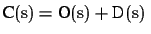Next: 7. Conclusion and Future Up: Toward Cost-Sensitive Modeling for Previous: 5. Experiments

# 6. Related Work

Several researchers and experts have pointed out the importance of using intrusion detection (and computer security in general) as a means of risk management [8,3,19]. Our work in cost-sensitive modeling for IDSs has benefited from their insightful analysis and extensive real-world experiences.

As discussed throughout the papers, our work draws from research in computer security assessment and intrusion taxonomies. In particular, Glaseman et al. discussed a model for evaluating the total expected cost in using a security system s as, where O(s) is the operational cost of s and D(s) is the expected loss . D(s) is calculated by summing the products of exposed value and the probability of safeguard failure over all possible threats. This model is similar to our cost model for IDSs, as defined in Equation 1. However, our definition of consequential cost allows cost-based optimization strategies to be explored because it includes the response cost and models its relationship with damage cost.

Credit card fraud detection and cellular phone fraud detection are closely related to intrusion detection because they also deal with detecting abnormal behavior. Both of these applications are motivated by cost-saving and therefore use cost-sensitive modeling techniques. In credit card fraud detection, for example, the cost factors include operation cost, the personnel cost of investigating a potentially fraudulent transaction (known as challenge cost), and loss (damage cost). If the dollar amount of a suspected transaction is lower than the challenge cost, the transaction is authorized and the credit card company will take the potential loss. Since the cost factors in fraud detection can be folded into dollar amounts, the cost-sensitive analysis and modeling tasks are much more simple than in intrusion detection.

Cost-sensitive modeling is an active research area in data mining and machine learning because of the demand from application domains such as medical diagnosis and fraud and intrusion detection. Several techniques have been proposed for building models optimized for given cost metrics. In our research we study the principles behind these general techniques and develop new approaches according to the cost models specific to IDSs.Next: 7. Conclusion and Future Up: Toward Cost-Sensitive Modeling for Previous: 5. Experiments